# 数据结构与算法——平衡二叉树（AVL树）

## 二叉排序树存在的问题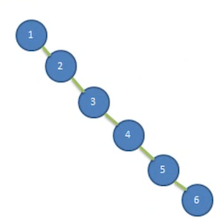1. 左子树全部为空，从形式上看，更像一个单链表

2. 插入速度没有影响

3. 但查询速度明显降低

因为需要依次比较，不能利用二叉排序树的折半优势。而且每次都还要比较左子树，可能比单链表查询速度还慢。

## 基本介绍

• 红黑树
• AVL（算法）
• 替罪羊树
• Treap
• 伸展树1. 是平衡二叉树：

• 左子树高度为 2
• 右子树高度为 1

他们差值为 1

2. 也是平衡二叉树

3. 不是平衡二叉树

1. 左子树高度为 3
2. 右子树高度为 1

他们差值为 2，所以不是

## 单旋转（左旋转）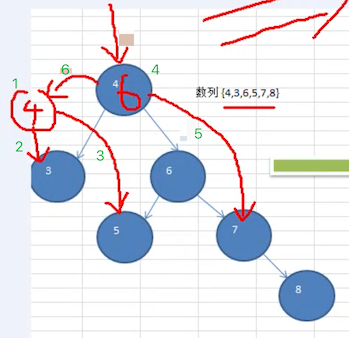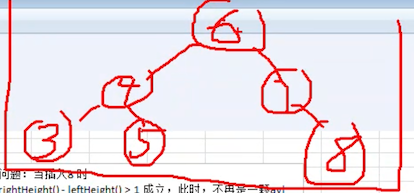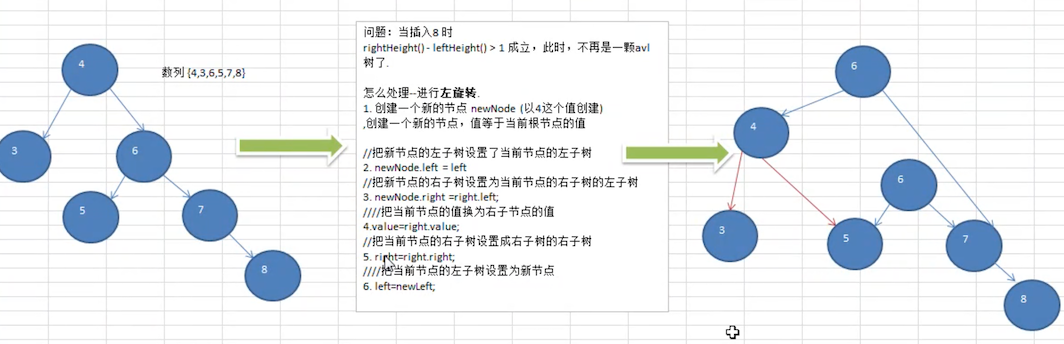1. 创建一个新的节点 newNode，值等于当前 根节点 的值（上图根节点为 4）

2. 把新节点的 左子树 设置为当前节点（根节点）的 左子树

newNode.left = this.left

3. 把新节点的 右子树 设置为当前节点(根节点)的 右子树 的 左子树

newNode.right = this.right.left

4. 当前节点(根节点) 的值换为 右子节点 的值

this.value = this.right.value

5. 当前节点（根节点） 的右子树设置为 右子树的右子树(按上图的话就是 7)

this.right = this.right.right

6. 当前节点(根节点) 的左子树设置为新节点newNode

this.left = this.newNode


：左图是调整前，右图是调整后。注意调整前的 6 那个节点，调整之后，没有节点指向他了。也就是说，遍历的时候它是不可达的。那么将会自动的被垃圾回收掉。

### 树高度计算

1. 这颗树的整体高度
2. 左子树的高度
3. 右子树的高度
public class AvlTreeTest {
/**
* 树高度测试
*/
@Test
public void heightTest() {
AvlTree tree = new AvlTree();
int[] arr = {4, 3, 6, 5, 7, 8};
for (int i = 0; i < arr.length; i++) {
}
tree.infixOrder();
System.out.println("树高度：" + tree.root.height());   // 4
System.out.println("左树高度：" + tree.root.leftHeight());  // 1
System.out.println("右树高度：" + tree.root.rightHeight()); // 3
}
}

/**
* 排序二叉树
*/
class AvlTree {
Node root;

public Node getRoot() {
return root;
}
}

/**
* 节点
*/
class Node {
/**
* 以当前节点为基础：计算出它包含它子树的所有高度
*
* @return
*/
public int height() {
/*
这里使用了递归：返回了左右子树中，最高的那一个数值。
递归原理：第一个开始统计的时候，一定是一个叶子节点
根据这个逻辑：叶子节点的 Math.max(0,0) = 0  看下面代码
因为当前节点算一层，所以 + 1;

返回到上一层的时候，至少是这样：Math.max(1,0) = 1
然后把自己本身的层 +1。 以此类推，返回到根节点的时候，就拿到了从包含根节点的树的高度
所以这个 +1 是精髓所在
*/
return Math.max(
(left == null ? 0 : left.height()),
(right == null ? 0 : right.height())
) + 1;
}

/**
* 计算左子树的高度
*
* @return
*/
public int leftHeight() {
if (left == null) {
return 0;
}
// 如果从根节点开始的话
// 其实它从中间分开，左侧就有很多的小树
// 所以还是要计算左右树的高度，返回一个最大的值，只不过是开始节点变化了
return left.height();
}

/**
* 计算右子树的高度，与上面的计算左子树同理
*
* @return
*/
public int rightHeight() {
if (right == null) {
return 0;
}
return right.height();
}
}


3
4
5
6
7
8



### 旋转

1. 每次添加完一个节点后，就需要检查树的高度

2. 满足 右子树高度 - 左子树高度 > 1，那么一定满足下面的条件：

①左子树高度为 1

②右子树高度为 3

也就是符合这张图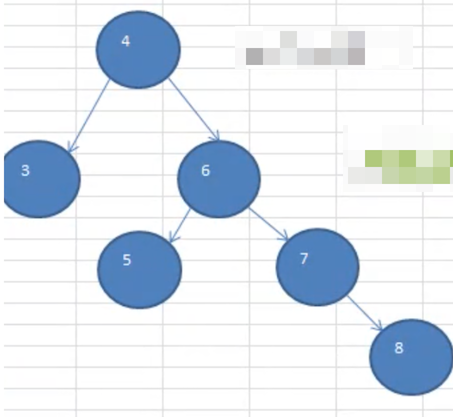class Node {
/**
* 添加节点：按照排序二叉树的要求添加
*
* @param node
*/
if (node == null) {
return;
}
// 如果添加的值小于当前节点，则往左走
if (node.value < value) {
// 左节点为空，则直接挂在上面
if (left == null) {
left = node;
} else {
// 否则继续往下查找
}
} else {
// 往右走
if (right == null) {
right = node;
} else {
}
}

// 旋转的时候有以下规则
// 每添加一个节点之后：检查树的高度是否平衡
//      如果右子树高度 - 左子树高度 > 1，则左旋转
//      也就是说：每次旋转的层只涉及到 4 层(对照笔记上的图示理解)
if (rightHeight() - leftHeight() > 1) {
leftRotate();
}
}

/**
* 以当前节点为根节点，进行左旋转
*/
public void leftRotate() {
// 1. 创建一个新的节点 newNode，值等于当前 根节点 的值
Node newNode = new Node(value);
// 2. 把 新节点的 左子树 设置为当前节点的左子树
newNode.left = left;
// 3. 把 新节点的 右子树 设置为当前节点的 右子树的左子树
newNode.right = right.left;
// 4. 把 当前节点的值，替换为 右子树 节点的子
value = right.value;
// 5. 把 当前节点 的 右节点 设置为 右子树的右子树
right = right.right;
// 6. 把 当前节点 的 左节点 设置为 新节点
left = newNode;
}
}


    /**
* 左旋转测试
*/
@Test
public void leftRotatedTest() {
AvlTree tree = new AvlTree();
int[] arr = {4, 3, 6, 5, 7, 8};
for (int i = 0; i < arr.length; i++) {
}
tree.infixOrder();
System.out.println("树高度：" + tree.root.height());   // 3
System.out.println("左树高度：" + tree.root.leftHeight());  // 2
System.out.println("右树高度：" + tree.root.rightHeight()); // 2
}


3
4
5
6
7
8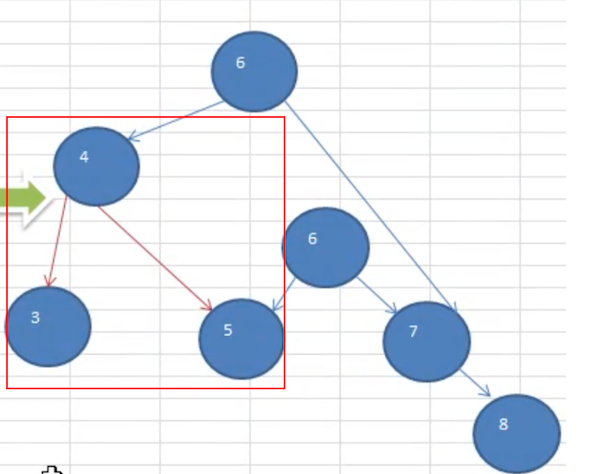1. 不用创建新节点
2. 直接将 node 节点下沉
3. 更改 node 的 right 节点为 right.left
4. 更改 right.left = node

## 右旋转

• 左旋转：右 - 左 > 1，把右边的往左边旋转一层
• 右旋转：左 - 右 > 1，把左边的往右边旋转一层

1. 创建一个新的节点 newNode，值等于当前 根节点 的值（以 4 创建）

2. 把新节点的 右子树 设置为当前节点（根节点）的 右子树

newNode.right = right

3. 把新节点的 左子树 设置为当前节点（根节点）的 左子树的右子树

newNode.left = left.right

4. 当前节点（根节点） 的值换为 左子节点 的值

value = left.value

5. 当前节点 （根节点）的左子树设置为 左子树的左子树

left = left.left

6. 当前节点 的右子树设置为新节点

right = newNode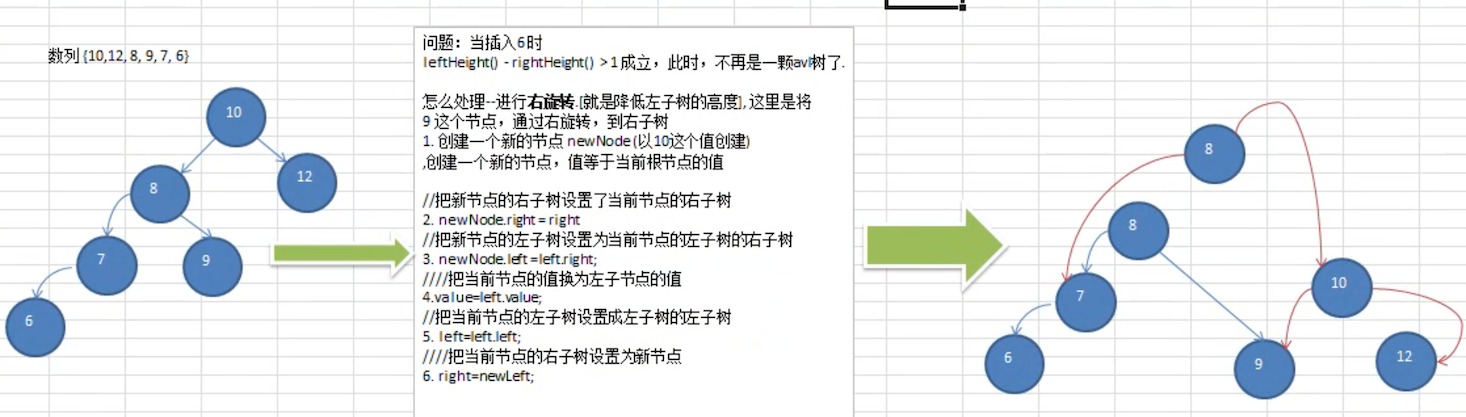class Node {
/**
* 添加节点：按照排序二叉树的要求添加
*
* @param node
*/
if (node == null) {
return;
}
// 如果添加的值小于当前节点，则往左走
if (node.value < value) {
// 左节点为空，则直接挂在上面
if (left == null) {
left = node;
} else {
// 否则继续往下查找
}
} else {
// 往右走
if (right == null) {
right = node;
} else {
}
}

// 旋转的时候有以下规则
// 每添加一个节点之后：检查树的高度是否平衡
//      如果右子树高度 - 左子树高度 > 1，则左旋转
//      也就是说：每次旋转的层只涉及到 4 层(对照笔记上的图示理解)
if (rightHeight() - leftHeight() > 1) {
leftRotate();
return;
}

if (leftHeight() - rightHeight() > 1) {
rightRotate();
}
}
/**
* 以当前节点为根节点，进行右旋转
*/
public void rightRotate() {
// 1. 创建一个新的节点 newNode，值等于当前 根节点 的值
Node newNode = new Node(value);
// 2. 把 新节点的 右子树 设置为当前节点的右子树
newNode.right = right;
// 3. 把 新节点的 左子树 设置为当前节点的 左子树的右子树
newNode.left = left.right;
// 4. 把 当前节点的值，替换为 左子树 节点的子
value = left.value;
// 5. 把 当前节点 的 左节点 设置为 左子树的左子树
left = left.left;
// 6. 把 当前节点 的 右节点 设置为 新节点
right = newNode;
}
}


    /**
* 右旋转测试
*/
@Test
public void rightRotatedTest() {
AvlTree tree = new AvlTree();
int[] arr = {10, 12, 8, 9, 7, 6};
for (int i = 0; i < arr.length; i++) {
}
tree.infixOrder();
System.out.println("树高度：" + tree.root.height());   // 3
System.out.println("左树高度：" + tree.root.leftHeight());  // 2
System.out.println("右树高度：" + tree.root.rightHeight()); // 2
System.out.println("当前根节点：" + tree.root); // 8
}


6
7
8
9
10
12



## 双旋转

int[] arr ={10,11,7,6,8,9}
int[] arr ={2,1,6,5,7,3}


    /**
* 不能通过单旋转解决的场景
*/
@Test
public void notLeftOrRightRotatedTest() {
AvlTree tree = new AvlTree();
int[] arr = {10, 11, 7, 6, 8, 9};
for (int i = 0; i < arr.length; i++) {
}
tree.infixOrder();
System.out.println("树高度：" + tree.root.height());   // 4
System.out.println("左树高度：" + tree.root.leftHeight());  // 1
System.out.println("右树高度：" + tree.root.rightHeight()); // 3
System.out.println("当前根节点：" + tree.root); // 7
}


6
7
8
9
10
11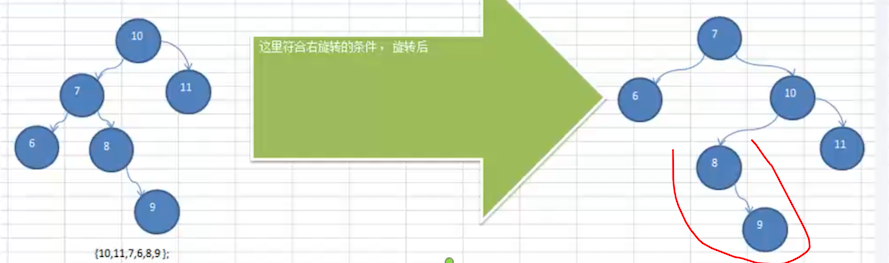1. 先将 7 这个节点作为 root 节点，进行左旋转
2. 再将原始的 root 节点进行右旋转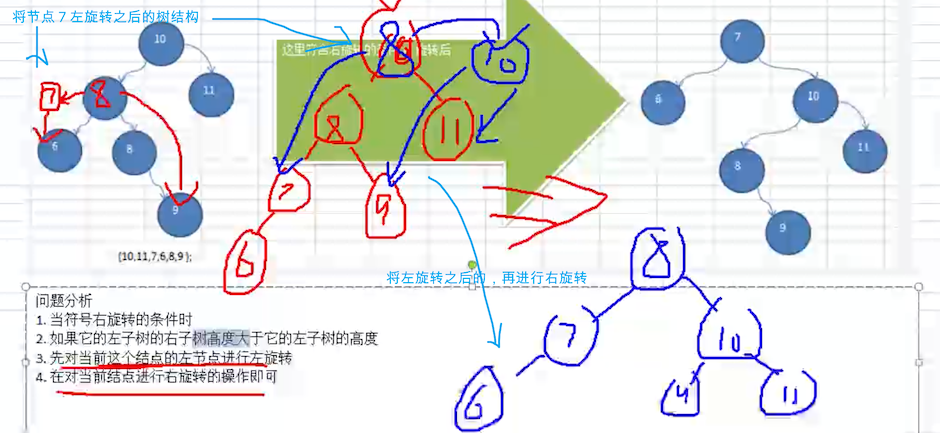1. 右旋转：
• root 的 left 左子树高度 大于 右子树高度
• 右旋转的时候，会将 left.right 旋转到 right.left 节点上
2. 左旋转：
• root 的 right 右子树高度 大于 左子树高度
• 左旋转的时候，会将 right.left 旋转到 left.right 上。

    /**
* 添加节点：按照排序二叉树的要求添加
*
* @param node
*/
if (node == null) {
return;
}
// 如果添加的值小于当前节点，则往左走
if (node.value < value) {
// 左节点为空，则直接挂在上面
if (left == null) {
left = node;
} else {
// 否则继续往下查找
}
} else {
// 往右走
if (right == null) {
right = node;
} else {
}
}

// 旋转的时候有以下规则
// 每添加一个节点之后：检查树的高度是否平衡
//      如果右子树高度 - 左子树高度 > 1，则左旋转
//      也就是说：每次旋转的层只涉及到 4 层(对照笔记上的图示理解)
// 						  小旋转的时候：只涉及到 3 层，旋转的时候，最多操作了当前节点和左右节点，所以不会导致 NPE 问题,这一点一定要明白
if (rightHeight() - leftHeight() > 1) {
// 当 右节点的：左子树高度 大于 右子树的高度时，将 right 节点进行 右旋转
if (right != null && right.leftHeight() > right.rightHeight()) {
right.rightRotate();
}
leftRotate();
return;
}

if (leftHeight() - rightHeight() > 1) {
// 当 左节点的：右子树高度 大于 左子树的高度时，将 left 节点进行左旋转
if (left != null && left.rightHeight() > left.leftHeight()) {
left.leftRotate();
}
rightRotate();
}
}


   /**
* 添加双旋转之后，之前测试不能旋转的数列进行测试
*/
@Test
public void doubleRotatedTest() {
AvlTree tree = new AvlTree();
int[] arr = {10, 11, 7, 6, 8, 9};
//        int[] arr ={2,1,6,5,7,3}
for (int i = 0; i < arr.length; i++) {
}
tree.infixOrder();
System.out.println("树高度：" + tree.root.height());
System.out.println("左树高度：" + tree.root.leftHeight());
System.out.println("右树高度：" + tree.root.rightHeight());
System.out.println("当前根节点：" + tree.root);
}


6
7
8
9
10
11

1
2
3
5
6
7



## 完整代码

public class AVLTreeDemo {

public static void main(String[] args) {
//int[] arr = {4,3,6,5,7,8};
//int[] arr = { 10, 12, 8, 9, 7, 6 };
int[] arr = {10, 11, 7, 6, 8, 9};
//创建一个 AVLTree对象
AVLTree avlTree = new AVLTree();
//添加结点
for (int i = 0; i < arr.length; i++) {
}

//遍历
System.out.println("中序遍历");
avlTree.infixOrder();

System.out.println("在平衡处理~~");
System.out.println("树的高度=" + avlTree.getRoot().height()); //3
System.out.println("树的左子树高度=" + avlTree.getRoot().leftHeight()); // 2
System.out.println("树的右子树高度=" + avlTree.getRoot().rightHeight()); // 2
System.out.println("当前的根结点=" + avlTree.getRoot());//8

}

}

// 创建AVLTree
class AVLTree {
private Node root;

public Node getRoot() {
return root;
}

// 查找要删除的结点
public Node search(int value) {
if (root == null) {
return null;
} else {
return root.search(value);
}
}

// 查找父结点
public Node searchParent(int value) {
if (root == null) {
return null;
} else {
return root.searchParent(value);
}
}

// 编写方法:
// 1. 返回的 以node 为根结点的二叉排序树的最小结点的值
// 2. 删除node 为根结点的二叉排序树的最小结点

/**
* @param node 传入的结点(当做二叉排序树的根结点)
* @return 返回的 以node 为根结点的二叉排序树的最小结点的值
*/
public int delRightTreeMin(Node node) {
Node target = node;
// 循环的查找左子节点，就会找到最小值
while (target.left != null) {
target = target.left;
}
// 这时 target就指向了最小结点
// 删除最小结点
delNode(target.value);
return target.value;
}

// 删除结点
public void delNode(int value) {
if (root == null) {
return;
} else {
// 1.需求先去找到要删除的结点 targetNode
Node targetNode = search(value);
// 如果没有找到要删除的结点
if (targetNode == null) {
return;
}
// 如果我们发现当前这颗二叉排序树只有一个结点
if (root.left == null && root.right == null) {
root = null;
return;
}

// 去找到targetNode的父结点
Node parent = searchParent(value);
// 如果要删除的结点是叶子结点
if (targetNode.left == null && targetNode.right == null) {
// 判断targetNode 是父结点的左子结点，还是右子结点
if (parent.left != null && parent.left.value == value) { // 是左子结点
parent.left = null;
} else if (parent.right != null && parent.right.value == value) {// 是由子结点
parent.right = null;
}
} else if (targetNode.left != null && targetNode.right != null) { // 删除有两颗子树的节点
int minVal = delRightTreeMin(targetNode.right);
targetNode.value = minVal;

} else { // 删除只有一颗子树的结点
// 如果要删除的结点有左子结点
if (targetNode.left != null) {
if (parent != null) {
// 如果 targetNode 是 parent 的左子结点
if (parent.left.value == value) {
parent.left = targetNode.left;
} else { // targetNode 是 parent 的右子结点
parent.right = targetNode.left;
}
} else {
root = targetNode.left;
}
} else { // 如果要删除的结点有右子结点
if (parent != null) {
// 如果 targetNode 是 parent 的左子结点
if (parent.left.value == value) {
parent.left = targetNode.right;
} else { // 如果 targetNode 是 parent 的右子结点
parent.right = targetNode.right;
}
} else {
root = targetNode.right;
}
}

}

}
}

// 添加结点的方法
if (root == null) {
root = node;// 如果root为空则直接让root指向node
} else {
}
}

// 中序遍历
public void infixOrder() {
if (root != null) {
root.infixOrder();
} else {
System.out.println("二叉排序树为空，不能遍历");
}
}
}

// 创建Node结点
class Node {
int value;
Node left;
Node right;

public Node(int value) {

this.value = value;
}

/**
* 以当前节点为基础：计算出它包含它子树的所有高度
*
* @return
*/
public int height() {
/*
这里使用了递归：返回了左右子树中，最高的那一个数值。
递归原理：第一个开始统计的时候，一定是一个叶子节点
根据这个逻辑：叶子节点的 Math.max(0,0) = 0  看下面代码
因为当前节点算一层，所以 + 1;

返回到上一层的时候，至少是这样：Math.max(1,0) = 1
然后把自己本身的层 +1。 以此类推，返回到根节点的时候，就拿到了从包含根节点的树的高度
所以这个 +1 是精髓所在
*/
return Math.max(
(left == null ? 0 : left.height()),
(right == null ? 0 : right.height())
) + 1;
}

/**
* 计算左子树的高度
*
* @return
*/
public int leftHeight() {
if (left == null) {
return 0;
}
// 如果从根节点开始的话
// 其实它从中间分开，左侧就有很多的小树
// 所以还是要计算左右树的高度，返回一个最大的值，只不过是开始节点变化了
return left.height();
}

/**
* 计算右子树的高度，与上面的计算左子树同理
*
* @return
*/
public int rightHeight() {
if (right == null) {
return 0;
}
return right.height();
}

//左旋转方法
private void leftRotate() {

//创建新的结点，以当前根结点的值
Node newNode = new Node(value);
//把新的结点的左子树设置成当前结点的左子树
newNode.left = left;
//把新的结点的右子树设置成带你过去结点的右子树的左子树
newNode.right = right.left;
//把当前结点的值替换成右子结点的值
value = right.value;
//把当前结点的右子树设置成当前结点右子树的右子树
right = right.right;
//把当前结点的左子树(左子结点)设置成新的结点
left = newNode;

}

//右旋转
private void rightRotate() {
Node newNode = new Node(value);
newNode.right = right;
newNode.left = left.right;
value = left.value;
left = left.left;
right = newNode;
}

// 查找要删除的结点

/**
* @param value 希望删除的结点的值
* @return 如果找到返回该结点，否则返回null
*/
public Node search(int value) {
if (value == this.value) { // 找到就是该结点
return this;
} else if (value < this.value) {// 如果查找的值小于当前结点，向左子树递归查找
// 如果左子结点为空
if (this.left == null) {
return null;
}
return this.left.search(value);
} else { // 如果查找的值不小于当前结点，向右子树递归查找
if (this.right == null) {
return null;
}
return this.right.search(value);
}

}

// 查找要删除结点的父结点

/**
* @param value 要找到的结点的值
* @return 返回的是要删除的结点的父结点，如果没有就返回null
*/
public Node searchParent(int value) {
// 如果当前结点就是要删除的结点的父结点，就返回
if ((this.left != null && this.left.value == value) || (this.right != null && this.right.value == value)) {
return this;
} else {
// 如果查找的值小于当前结点的值, 并且当前结点的左子结点不为空
if (value < this.value && this.left != null) {
return this.left.searchParent(value); // 向左子树递归查找
} else if (value >= this.value && this.right != null) {
return this.right.searchParent(value); // 向右子树递归查找
} else {
return null; // 没有找到父结点
}
}

}

@Override
public String toString() {
return "Node [value=" + value + "]";
}

// 添加结点的方法
// 递归的形式添加结点，注意需要满足二叉排序树的要求
if (node == null) {
return;
}

// 判断传入的结点的值，和当前子树的根结点的值关系
if (node.value < this.value) {
// 如果当前结点左子结点为null
if (this.left == null) {
this.left = node;
} else {
// 递归的向左子树添加
}
} else { // 添加的结点的值大于 当前结点的值
if (this.right == null) {
this.right = node;
} else {
// 递归的向右子树添加
}

}

// 旋转的时候有以下规则
// 每添加一个节点之后：检查树的高度是否平衡
//      如果右子树高度 - 左子树高度 > 1，则左旋转
//      也就是说：每次旋转的层只涉及到 4 层(对照笔记上的图示理解)
// 						  小旋转的时候：只涉及到 3 层，旋转的时候，最多操作了当前节点和左右节点，所以不会导致 NPE 问题,这一点一定要明白
if (rightHeight() - leftHeight() > 1) {
// 当 右节点的：左子树高度 大于 右子树的高度时，将 right 节点进行 右旋转
if (right != null && right.leftHeight() > right.rightHeight()) {
right.rightRotate();
}
leftRotate();
return;
}

if (leftHeight() - rightHeight() > 1) {
// 当 左节点的：右子树高度 大于 左子树的高度时，将 left 节点进行左旋转
if (left != null && left.rightHeight() > left.leftHeight()) {
left.leftRotate();
}
rightRotate();
}
}

// 中序遍历
public void infixOrder() {
if (this.left != null) {
this.left.infixOrder();
}
System.out.println(this);
if (this.right != null) {
this.right.infixOrder();
}
}

}

posted @ 2021-09-18 15:36  海绵寳寳  阅读(267)  评论(2编辑  收藏  举报